Vol 17, No 7 (2017) / Wang

The photometric monitoring of <em>γ</em>-ray-loud narrow-line Seyfert 1 galaxy 1H 0323+342 from 2006 to 2010

The photometric monitoring of γ-ray-loud narrow-line Seyfert 1 galaxy 1H 0323+342 from 2006 to 2010

Wang Fang1, 2, 3, 4, , Xiong Ding-Rong1, 3, 4, , Bai Jin-Ming1, 3, 4, Li Shao-Kun1, 3, 4, Wang Jian-Guo1, 3, 4

Yunnan Observatories, Chinese Academy of Sciences, Kunming 650216, China
University of Chinese Academy of Sciences, Beijing 100049, China
Key Laboratory for the Structure and Evolution of Celestial Objects, Chinese Academy of Sciences, Kunming 650216, China
Center for Astronomical Mega-Science, Chinese Academy of Sciences, Beijing 100012, China

† Corresponding author. E-mail: fwang@ynao.ac.cn xiongdingrong@ynao.ac.cn

Abstract: Abstract

1H 0323+342 is a γ-ray-loud narrow-line Seyfert 1 galaxy (NLS1). The variability mechanism of γ-ray-loud NLS1s remains unclear. We have observed 1H 0323+342 photometrically from 2006 to 2010 with a total of 41 nights of observations in order to constrain the variability mechanism. Intraday variabilities (IDVs) are detected on four nights. When considering the nights with time spans > 2 hours, the duty cycle is 28.3%. The average variability amplitude is 10.8% for IDVs and possibly variable nights. In the color–magnitude diagram, there are bluer-when-brighter chromatic trends for intraday and long-term timescales, which could be explained by the shock-in-jet model, and also could possibly be due to two distinct components or an accretion disk model.

Keywords: galaxies: active;galaxies: individual (1H 0323+342);galaxies: jets

1 Introduction

Narrow-line Seyfert 1 galaxies (NLS1s) are usually radio-quiet active galactic nuclei (AGNs). However, Yuan et al. () studied a comprehensive sample of 23 genuine radio-loud NLS1s with radio loudness larger than 100. Some of these objects show interesting radio to X-ray properties that are unusual for most previously known radio-loud NLS1 AGNs, but are reminiscent of blazars. They interpreted them as signatures of the postulated blazar nature, which likely possess at least moderately relativistic jets. Abdo et al. () first reported the discovery of high-energy γ-ray emission from the peculiar NLS1 PMN J0948+0022 (z = 0.5846) by the Large Area Telescope (LAT) onboard the Fermi Gamma-ray Space Telescope. Then Abdo et al. () further reported the discovery of γ-ray emission from three radio-loud NLS1s: PKS 1502+036, 1H 0323+342 and PKS 2004-447 with Fermi/LAT. They constructed the spectral energy distributions (SEDs) of the four γ-ray-loud NLS1s. The fitting to the SEDs revealed these emissions are from both the accretion disk and the jet.

Blazars can be classified as flat spectrum radio quasars (FSRQs) and BL Lacertae (BL Lac) objects. FSRQs have strong emission lines, while BL Lac objects have only very weak or non-existent emission lines. Objects with rest frame equivalent width ≥ 5 Å are classified as FSRQs (e.g., Urry & Padovani ; Scarpa & Falomo ). The same as that of blazars, the variability of γ-ray-loud NLS1s can be classified as long-term variability (LTV), short-term variability (STV) and intraday variability (IDV). LTVs range from months to years, and STVs range from days to weeks, even months (Gupta et al. ; Dai et al. ). The variations on timescales of tens of minutes or a few hours are IDVs. The variation amplitudes of IDVs are a few tenths of or hundredths of a magnitude (Wagner & Witzel ). Understanding the variability mechanism is important in understanding the nature of γ-ray-loud NLS1s. Variability can shed light on the radiation mechanism, and the physics and formation mechanism of jets.

1H 0323+342 is the nearest γ-ray-loud NLS1 (z = 0.061). Its V band magnitude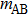is 15.0 (Paliya et al. ). Wang et al. () measured the black hole mass to be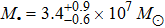using reverberation mapping. They calculated its Eddington ratio to be 0.15. Zhou et al. () found that its host galaxy exhibited an apparent one-armed spiral structure. Antón et al. (2008) investigated the host galaxy by the analysis of B and R images obtained with the 2.6 m Nordic Optical Telescope under good photometric conditions. Their study showed that the host galaxy had a ring-like morphology. Paliya et al. () constructed SEDs during different activity states and modeled them using a one-zone leptonic model. They argued that 1H 0323+342 possesses dual characteristics, akin to FSRQs as well as radio-quiet NLS1s. Paliya et al. () carried out optical flux monitoring observations of 1H 0323+342. They observed it on four nights. On one of the four nights, IDV was detected.

The γ-ray-loud NLS1s possess relativistic jets (Foschini , Liu et al. ). Whether their variability mechanism and the spectral properties are the same as those of blazars is still not clear. We have observed 1H 0323+342 photometrically from 2006 to 2010 with a total of 41 nights of observations in order to constrain the variability mechanism and spectral properties.

2 Observations and Data Reduction

1H 0323+342 was monitored in a photometric long-term monitoring project from 2006 January to 2010 March, with a total of 41 nights of observations. From 2006 January to 2008 January, the PI1024 TKB CCD photometric system attached to the 1.0 m Cassegrain reflecting telescope administered by Yunnan Observatories was used for observation. From 2008 September to 2009 January, the DW436 2048×2048 CCD attached to the same 1.0 m telescope was used. From 2009 January to 2010 March, the Princeton Instruments CCD installed on the 2.4 m telescope administered by Lijiang Observatory, Yunnan Observatories was used. The standard Johnson B, V, R and I band observations were performed. Different CCDs would lead to different quantum effects. Because we used differential photometry, the measured differential magnitudes were relative to the unchanged standards;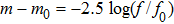,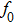was the standard flux, f was the source flux,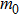was the standard magnitude and m was the source magnitude. When the CCD is in a linear response region, the quantum effects affect both f andwith the same scale. So, the differential magnitude is unaffected.

The images of the project were reduced using the zphot package. The zphot package invoked the IRAF daophot package to automatically perform data reduction. It first creates a template for the star field. Then for each image, it detects the positions of the target and comparison stars, and the positions are used as input for photometry. This procedure was repeated automatically until all images were reduced. The aperture of photometry was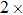full width at half maximum (FWHM). Adopting this aperture, the standard deviations of the standard stars were the smallest compared with other apertures in our test. Following Zhang et al. (, ), Fan et al. () and Bai et al. (), we obtained the source magnitude from two standard stars in the same image. The differential magnitude was obtained by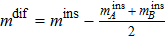, where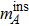and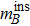are the instrumental magnitudes of the two standards. The two standards were the S1 (standard star B) and S3 (standard star A) in table 2 of Paliya et al. (). The error of the photometry on a certain night was the standard deviation of the differential magnitude between the two standard stars.

Date (UT) Band N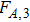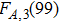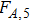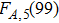V/N A%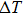(h)
(1) (2) (3) (4) (5) (6) (7) (8) (9) (10)
2006–09–21 I 16 3.31 5.67 0.49 6.70 N
2006–09–25 B 24 0.51 4.03 1.74 4.94 N
2006–09–25 V 22 1.33 4.32 2.77 5.09 N
2006–09–25 I 24 1.12 4.03 2.07 4.94 N
2006–11–14 I 21 1.32 4.46 2.15 5.18 N
2006–11–30 I 16 2.39 5.67 0.57 6.70 N
2006–12–01 V 18 0.44 5.06 1.89 6.36 N
2006–12–01 I 25 1.34 3.93 0.39 4.43 N
2006–12–02 I 16 0.53 5.67 1.08 6.70 N
2009–10–23 B 120 0.78 1.86 1.25 2.01 N
2009–10–23 V 120 1.21 1.86 1.13 2.01 N
2009–10–23 R 120 0.98 1.86 0.95 2.01 N
2009–10–23 I 120 2.80 1.86 4.26 2.01 V 17.28 2.17
2009–10–27 B 61 1.43 2.38 0.94 2.63 N
2009–10–27 V 61 0.92 2.38 1.12 2.63 N
2009–10–27 R 61 1.61 2.38 1.32 2.63 N
2009–10–27 I 61 3.24 2.38 3.29 2.63 V 8.81 0.47
2009–10–31 B 121 8.35 1.85 10.60 2.01 V 15.34 2.64
2009–10–31 V 121 11.39 1.85 13.86 2.01 V 13.28 3.17
2009–10–31 R 121 11.48 1.85 17.35 2.01 V 11.69 2.11
2009–10–31 I 121 11.24 1.85 15.62 2.01 V 12.61 2.05
2009–11–09 V 70 2.25 2.25 3.55 2.47 PV 7.43 1.45
2009–11–09 R 125 1.18 1.84 1.53 1.98 N
2009–11–09 I 34 0.79 3.21 0.61 3.75 N
2009–11–12 V 38 1.92 3.02 4.76 3.45 PV 6.85 0.42
2009–11–12 R 38 2.03 3.02 2.69 3.45 N
2009–11–12 I 38 1.62 3.02 1.81 3.45 N
2010–02–16 V 31 10.62 3.40 11.10 3.85 V 3.79 0.51
2010–02–16 R 36 1.23 3.09 2.06 3.50 N
2010–02–16 I 31 3.96 3.40 5.01 3.85 V 11.42 0.59

Notes: Column (1) is the date of observation, Col. (2) is the observed band, Col. (3) is the number of data points, Col. (4) is the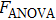for a group of three exposures, Col. (5) is the criticalwith 99% confidence level for a group of three exposures, Col. (6) is thefor a group of five exposures, Col. (7) is the criticalwith 99% confidence level for a group of five exposures, Col. (8) is the variability status (Whenexceeds the critical value(99) andexceeds the critical value(99), it is variable (V); when eitherexceeds(99) orexceeds(99), it is probably variable (PV); if neitherexceeds(99) norexceeds(99), it is non-variable (N).), Col. (9) is the variability amplitude, Col. (10) is the time separation between the point with highest flux and the point with lowest flux in the light curve.

Table 2 Results of IDV Observations of 1H 0323+342

After the differential magnitudes were obtained, in order to estimate the contamination of the host galaxy, we checked the aperture–differential magnitude relations. For all data from the 2.4 m telescope, the aperture–differential magnitude relations are given in Figure 1. The Pearson correlation coefficients of the B/V/R/I bands are −0.82, −0.82, −0.92, −0.96, respectively, i.e. the differential magnitudes had strong correlations with apertures. These correlations could be due to the effect of the host galaxy. During one night, the value of FWHM is almost constant, but the small change in seeing can still cause changes to the FWHM and aperture radius. The different aperture radius can include different host galaxy components. For a longer-term timescale, different nights or periods are likely to have larger changes in seeing, which may cause larger false variability in objects with a prominent host galaxy (Xiong et al. ). The results from León Tavares et al. () and Liu et al. () indicated that the host galaxy effect for the object could not be neglected. For the 1 m telescope, the Pearson correlation coefficients of the B/V/R/I bands were −0.73, −0.57, −0.96, −0.86, respectively. So, the corrections for the host galaxy effect were applied to the differential magnitudes. In order to correct the host galaxy effect, we used the aperture–differential magnitude relations to correct the differential magnitude to reach the same aperture size. First, linear fits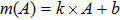to the aperture–differential magnitude correlation were performed. Here, m is the differential magnitude and A is the aperture size in arcminute. Then, the magnitudes were corrected by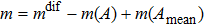, while A was the aperture size for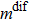and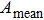was the mean aperture of each band used in the 1 m telescope and the 2.4 m telescope.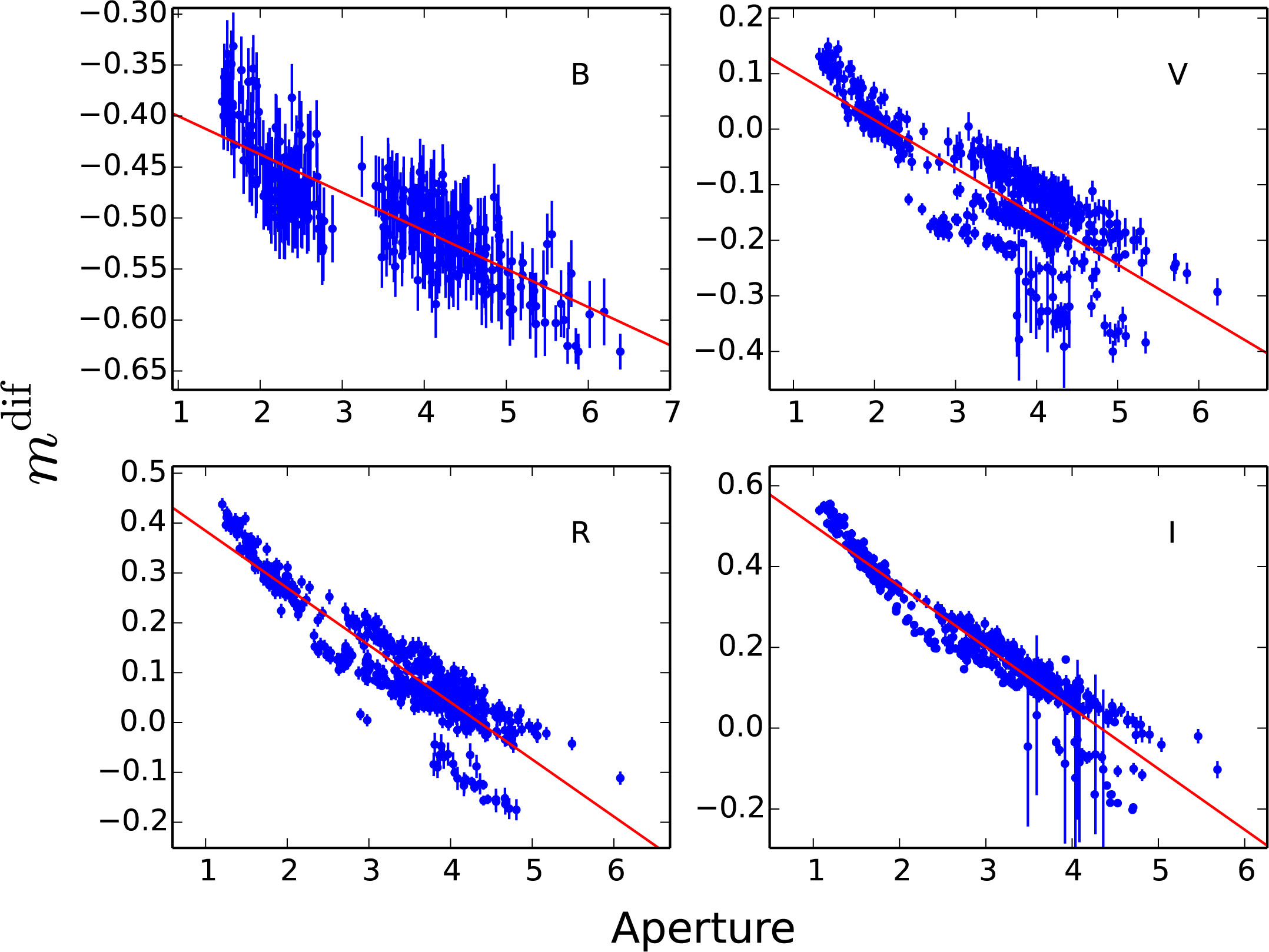Fig. 1 The aperture–differential magnitude relation for the 2.4 m telescope data.

Table 1 is the observation log for nights with number of observations N > 15. There were two different exposure modes. One was first exposing a band several times, then another band (AAA…BBB…). The other was exposing the bands in turn (ABCDABCD…). For data observed using the 1.0 m telescope, the first mode was adopted. For data observed by the 2.4 m telescope, both modes were adopted. When calculating the color, we wrote a program which could pair the data in different bands. This program removed data pairs which have time separation >10 minutes. A separation of 10 minutes was used because it should satisfy the simultaneity criteria for variability between the involved bands (de Diego ). So, the data collected by the second mode were mainly used to analyze the color.

Date (UT) Telescope (m) Band N Exposure (s) Duration (h)
(1) (2) (3) (4) (5) (6)
2006–09–21 1 I 16 120 3.46
2006–09–25 1 B 24 250 3.33
2006–09–25 1 V 22 150 3.14
2006–09–25 1 I 24 90 3.14
2006–11–14 1 I 21 90 1.70
2006–11–30 1 I 16 120 0.65
2006–12–01 1 V 18 180 1.48
2006–12–01 1 I 25 90 2.12
2006–12–02 1 I 16 100 1.78
2009–10–23 2.4 B 120 20 2.69
2009–10–23 2.4 V 120 15 2.69
2009–10–23 2.4 R 120 10 2.69
2009–10–23 2.4 I 120 10 2.69
2009–10–27 2.4 B 61 20 1.36
2009–10–27 2.4 V 61 15 1.36
2009–10–27 2.4 R 61 10 1.36
2009–10–27 2.4 I 61 10 1.36
2009–10–31 2.4 B 121 30 3.56
2009–10–31 2.4 V 121 25 3.56
2009–10–31 2.4 R 121 15 3.56
2009–10–31 2.4 I 121 10 3.56
2009–11–09 2.4 V 70 20 2.05
2009–11–09 2.4 R 125 10 2.08
2009–11–09 2.4 I 34 10 0.86
2009–11–12 2.4 V 38 20 0.65
2009–11–12 2.4 R 38 10 0.65
2009–11–12 2.4 I 38 10 0.65
2010–02–16 2.4 V 31 60 1.67
2010–02–16 2.4 R 36 40 1.97
2010–02–16 2.4 I 31 40 1.70

Notes: Column (1) is the date of observation, Col. (2) is the telescope used, Col. (3) is the observed band, Col. (4) is the number of exposures, Col. (5) is the median of exposure time and Col. (6) is the observation duration.

Table 1 Observation Log

3 Results

The F-test and C-test are usually applied in variability analysis. However, because we have corrected the host galaxy, and the variance/standard deviation of source-star A/B could not be directly calculated, we do not use the F-test or C-test in this paper. Analysis of Variance (ANOVA) is a powerful and robust estimator for IDVs (de Diego ). It divides the light curve into many groups, with each group having an average value. It calculates the variance for the average values of each group. It also calculates the variance within each group and compares the two variances (de Diego , Appendix A3). It does not rely on error measurement but derives the expected variance from subsamples of the data. A group of three exposures and a group of five exposures were used in binning the exposures on account of the exposure times. de Diego () reported that ANOVA is a powerful and robust estimator for microvariations. They suggested that performing the test for bins of size five might be enough to ensure detection on a wide range of timescales and amplitudes. However, if the bin size is smaller, the time resolution is better, and the number of groups increases, which could increase the statistical significance. In consideration of the time resolution and number of groups, we also used the three-exposure group. These methods were applied to nights with more than 15 exposures. If the number of exposures in the last group was less than three or five, it was merged with the previous group (Xiong et al. ). The computedvalues were compared with the critical values,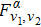, where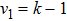(k is the number of groups),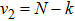(N is the number of measurements) and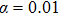is the significance level (Hu et al. ). If thevalue is larger than the critical value, the null hypothesis (no variability) is discarded, i.e., the source is variable at a confidence level of 99%. The light curves were considered variable when thefor a group of three exposures and a group of five exposures both exceeded the critical values. These results are shown in Table 2.

The IDV light curves are shown in Figure 2. The nights with number of exposures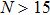are listed in Table 2. In Cols. (4) and (5), the values listed are the ANOVA results of a group of three exposures. In Cols. (6) and (7), the values listed are the ANOVA results of a group of five exposures. In Col. 9 of Table 2, the variability amplitudes (Amp) are calculated by Heidt & Wagner ()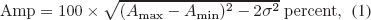where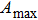and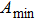are the maximum and minimum magnitude, respectively, of the light curve of the night being considered;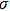is the standard deviation of the differential magnitudes between comparison star A and comparison star B on that night. The average variability amplitude is 10.8% for variable and possibly variable nights.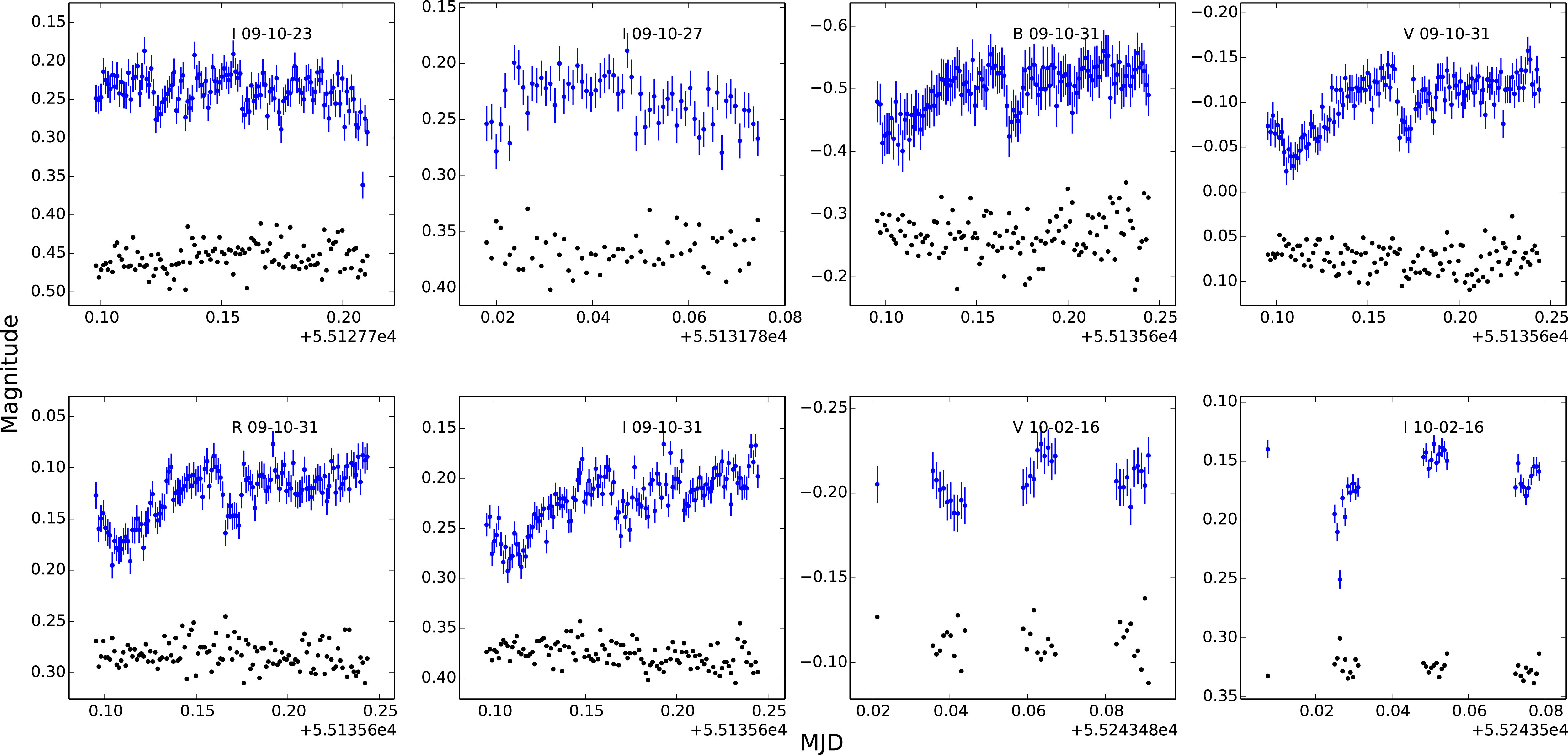Fig. 2 The IDV light curves. The blue dots with error bars are the light curves for 1H 0323+342. The black dots indicate the variability of the standards. The light curves of the standards are offset to avoid overlapping with the light curves of 1H 0323+342.

The duty cycle (DC) of 1H 0323+342 is calculated as (Romero et al. ; Stalin et al. ; Hu et al. ; Xiong et al. )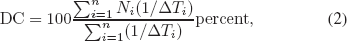where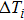is the duration of the monitoring session of the ith night.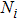will be set to 1 if IDV is detected, otherwise. DC is the probability for a source to have IDV. When calculating the value of DC, we only considered nights with more than 15 points and with durations longer than two hours. The calculated DC was 28.3%, which could be underestimated because of the short observation durations of the nights. It increased to 37.6% if the daily light curves satisfying any of the twocriteria were included.

Paliya et al. () also detected IDVs in the light curve of 1H 0323+342. Using the C-statistics to classify the variability nature of three γ-ray-loud NLS1s, they obtained a DC of IDV of57% on average. However, if only considering the observations of 1H 0323+342 in their paper, there was IDV observed on one night of a total of four nights using C-statistics, and IDVs observed on two nights using F-statistics (i.e., a DC of25%-50%). Our DC result is 28.3%, which could be underestimated because of the short observation durations of the nights. It increases to 37.6% if the daily light curves satisfying any of the twocriteria are included. Our DC result is consistent with theirs.

3.2 Long-term Optical Variability

Because the aperture–differential magnitude correlations are different for different telescopes, we have applied different corrections to different telescopes. So, we process the long-term light curves of the 1 m telescope and the 2.4 m telescope separately. The long-term light curves are shown in Figures 3 and 4. From them, 1H 0323+342 exhibits variability both on short and long timescales. We calculate the overall magnitude changes as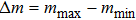. The overall magnitude changes for the 1m telescope data are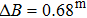,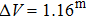,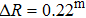and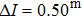. The overall magnitude changes for 2.4 m telescope data are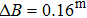,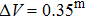,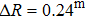and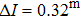.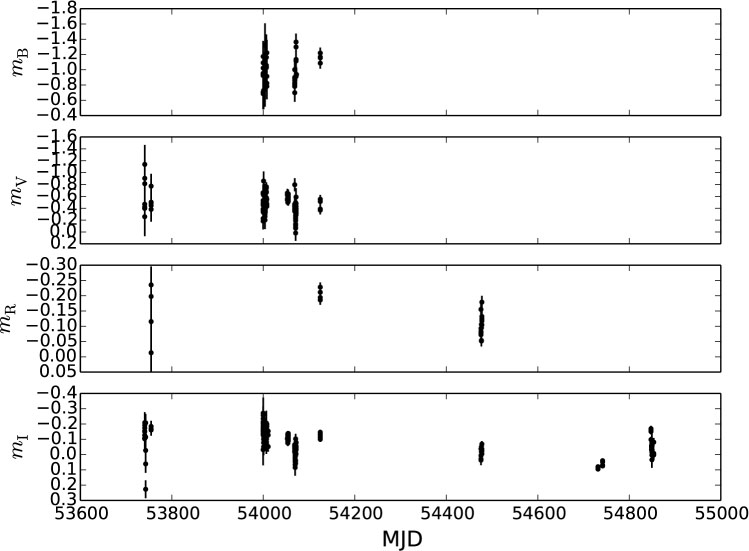Fig. 3 The long-term light curves of 1H 0323+342 in the B, V, R and I bands of the 1 m telescope.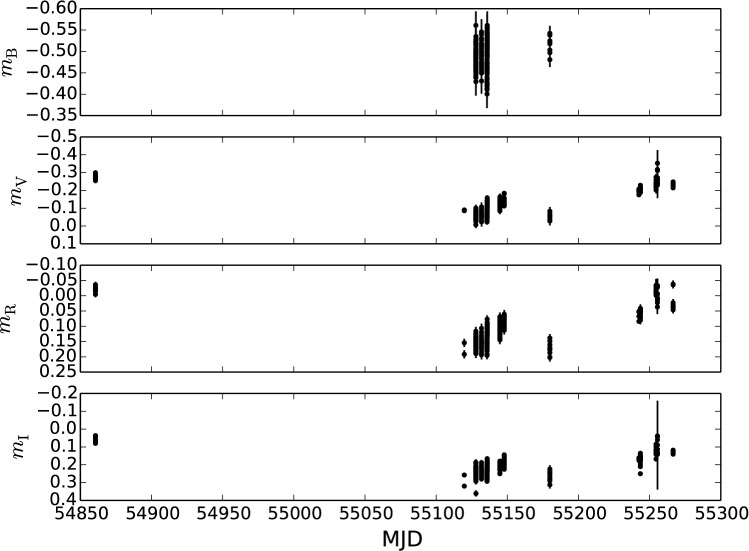Fig. 4 The long-term light curves of 1H 0323+342 in the B, V, R and I bands of the 2.4 m telescope.
3.3 Correlations between Colors and Magnitudes

In order to explore the spectral property, the correlations between colors and magnitudes are studied. Because both of the two bands used to calculate the color can be plotted as the x-axis, their averages are used so that the information of both bands is used (e.g., Dai et al. ). For intraday timescales, we only analyze the nights with number of VR colors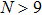. The correlations between VR colors and the average magnitudes of V and R are plotted in Figure 5. The results of correlations between the VR colors and the magnitudes are given in Table 3.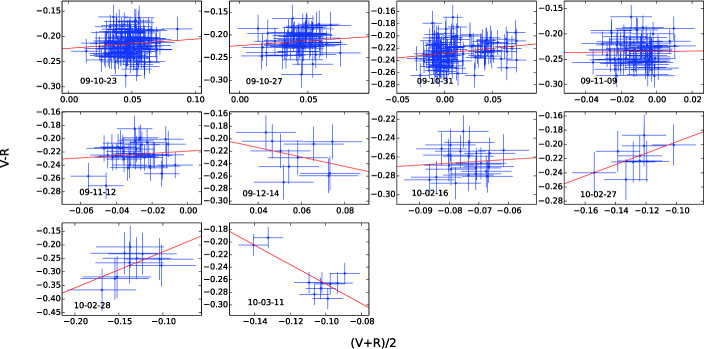Fig. 5 The V − R color–magnitude diagrams for individual days.
Date (UT) Bands r P
2009–10–23 VR 0.10 2.976e–01
2009–10–27 VR 0.11 3.967e–01
2009–10–31 VR 0.21 1.862e–02
2009–11–09 VR 0.02 8.958e–01
2009–11–12 VR 0.11 5.126e–01
2009–12–14 VR –0.32 3.723e–01
2010–02–16 VR 0.09 6.920e–01
2010–02–27 VR 0.59 7.546e–02
2010–02–28 VR 0.58 7.985e–02
2010–03–11 VR –0.80 5.092e–03
2009–10–27 to 2009–12–14 B — V 0.08 2.992e–01
2009–10–27 to 2009–12–14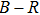0.26 3.050e–04
2009–10–27 to 2009–12–14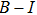0.22 2.056e–03
2009–10–23 to 2010–03–11 VR 0.43 8.853e–23
2009–10–23 to 2010–03–11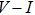0.48 1.264e–25
2009–10–23 to 2010–03–11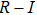0.26 2.571e–08

Table 3 The Pearson Correlation Coefficients and P value Results

From Figure 5 and Table 3, we can see that for individual nights with Pearson coefficient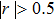, two nights exhibit the bluer-when-brighter (BWB) chromatic trend (2010–02–27 and 2010–02–28), and one night shows the redder-when-brighter (RWB) chromatic trend. However, we do not consider the RWB night as having reliable results because there are only two data points in the top-left corner. Therefore, two nights show a good BWB chromatic trend on intraday timescales.

Because the host galaxy corrections are different for the 1 m and 2.4 m telescopes and the data from the 1 m telescope are collected using the first exposure mode described in Section 2, we only plot the color–magnitude correlations for all the 2.4 m telescope data (Fig. 6). The Pearson correlation coefficients and P values of short-term (2009–10–27 to 2009–12–14) and long-term (2009–10–23 to 2010–03–11) color–magnitude correlations are also listed in Table 3. The color–magnitude correlations all show BWB trends for short and long timescales. For short-term timescales, the color–magnitude correlations are weak, with Pearson coefficients < 0.3. For VR/VI color–magnitude correlations of long-term timescales, the Pearson coefficients > 0.4. The Pearson coefficient of the RI color–magnitude correlation for the long-term timescale is 0.26. Therefore, for VR/VI color–magnitude correlations, the BWB trends are found in long-term timescales (2009–10–23 to 2010–03–11).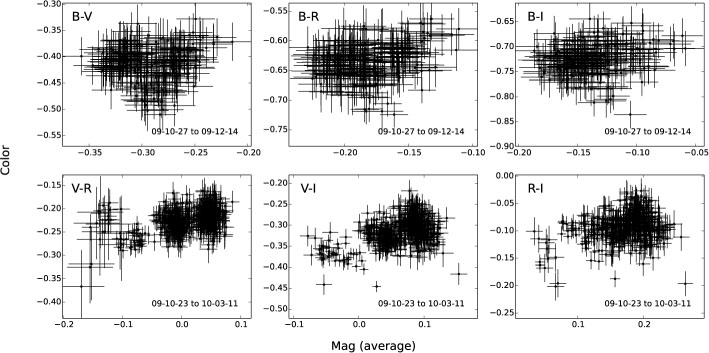Fig. 6 The color–magnitude diagrams for longer timescales. The magnitudes are the average of the two bands used to calculate the colors.

4 Discussion and Conclusions

León Tavares et al. () decomposed the surface profile of 1H 0323+342 into a point source plus a Sérsic profile. The point source was from the AGN emission. The Sérsic profile was the host galaxy. They found that the host galaxy took up 45%–73% of the total emission from the B band to the J band. Liu et al. studied the variation of 1H 0323+342. A difference image subtraction technique (Choi et al. ) was used to subtract the host galaxy. They also plotted the FWHM–differential magnitude diagram and found that the host galaxy effect could not be neglected. For our results on differential photometry, we still found that there are strong correlations between apertures and differential magnitudes. So, we applied the host galaxy correction described in Section 2.

For intraday and long-term timescales, the BWB chromatic trends are found. Compared with the results of the non-corrected host galaxy effect, after correcting the host galaxy effect, the results tend more to BWB chromatic trends. The host galaxy contribution is a non-variable redder component. So after correcting the host galaxy effect, the relations of color and magnitude tend more to BWB chromatic trends. Usually, blazars become BWB (Ghisellini et al. ; Fan et al. ; Fan & Lin ; Gu et al. ; Xiong et al. ). The BWB behavior is most likely to support the shock-in-jet model. According to the shock-in-jet model, as the shock propagates down the jet, it strikes a region with a large electron population. Radiation at different visible colors is produced at different distances behind the shocks. High-energy photons from the synchrotron mechanism typically emerge sooner and closer to the shock front than the lower frequency radiation, thus causing color variations (Agarwal & Gupta ). The BWB trend can also be explained in that there are two components contributing to the total emission. One variable component has a flatter spectrum and the other stable component has a steeper spectrum. Bian et al. () found that spectra of half of the QSOs appear redder during their brighter phases for their sample with redshift up to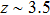. Guo & Gu () found that most quasars (~ 94%) show the BWB trend. Sun et al. () further discovered that the variable emission at shorter timescales has a stronger BWB trend than that at longer timescales. The thermal accretion disk fluctuation model is favored in their study. The fluctuations in accretion disk occur first in the inner disk region, then propagate outward, so BWB occurs. When the thermal radiation of disk dominates the total flux, an accretion disk model can explain the BWB trend (Gu & Li ; Li & Cao ; Liu et al. ). Therefore, like blazars, the BWB trends for 1H 0323+342 on intraday timescales could be explained by the shock-in-jet model, and also possibly be due to two distinct components or the accretion disk model. For long timescales, weaker BWB trends are found, which could be due to Doppler factor variations on a convex spectrum and superposition of different components (Gu et al. ; Xiong et al. ).

Due to the sampling rate of the long-term light curve being sparse, we mainly focus on the IDV. The mechanism for the IDV could be the ‘shock in jet’ model, in which the shock propagates down the jet, sweeping emitting regions. If the emitting regions have large intrinsic changes (magnetic field, particle velocity/distribution, a large number of new particles injected), then we could see a large flare on a very short variability timescale (Xiong et al. ). The mechanism could also be the ‘jets in a jet’ model (Narayan & Piran ). In this model, many sub-jets in the jet propagate along curved paths. When one sub-jet moves towards the observer, a flare reaches its peak flux; then this sub-jet deviates from the line of sight and this flare fades away. After some time, new sub-jets move towards the observer and new flares occur.

In conclusion, 1H 0323+342 was observed photometrically from 2006 to 2010 with a total of 41 nights of observations in order to constrain the variability mechanism. IDVs were found in the light curves. The calculated DC was 28.3%. In the color–magnitude diagram, there were BWB chromatic trends for the intraday and long-term timescales, which could be explained by the shock-in-jet model, and also possibly be due to two distinct components or the accretion disk model.

 Abdo A. A. Ackermann M. Ajello M. et al. 2009a ApJ 699 976 Abdo A. A. Ackermann M. Ajello M. et al. 2009b ApJ 707 L142 Agarwal A. Gupta A. C. 2015 MNRAS 450 541 Antón S. Browne I. W. A. Marchã M. J. 2008 A&A 490 583 Bai J. M. Xie G. Z. Li K. H. Zhang X. Liu W. W. 1998 A&AS 132 83 Bian W.-H. Zhang L. Green R. Hu C. 2012 ApJ 759 88 Choi Y. Gibson R. R. Becker A. C. et al. 2014 ApJ 782 37 Dai B.-Z. Zeng W. Jiang Z.-J. et al. 2015 ApJS 218 18 de Diego J. A. 2010 AJ 139 1269 Fan J. H. Xie G. Z. Pecontal E. Pecontal A. Copin Y. 1998 ApJ 507 173 Fan J. H. Lin R. G. 1999 ApJS 121 131 Fan J. H. Kurtanidze O. Liu Y. et al. 2014 ApJS 213 26 Foschini, L. 2012, in Proceedings of Nuclei of Seyfert Galaxies and QSOs - Central Engine & Conditions of Star Formation (Seyfert 2012) (http://pos.sissa.it/cgi-bin/reader/conf.cgi?confid=169) Ghisellini G. Villata M. Raiteri C. M. et al. 1997 A&A 327 61 Gu M. F. Lee C.-U. Pak S. Yim H. S. Fletcher A. B. 2006 A&A 450 39 Gu M. F. Li S.-L. 2013 A&A 554 A51 Guo H. Gu M. 2016 ApJ 822 26 Gupta A. C. Fan J. H. Bai J. M. Wagner S. J. 2008 AJ 135 1384 Heidt J. Wagner S. J. 1996 A&A 305 42 Hu S. M. Chen X. Guo D. F. Jiang Y. G. Li K. 2014 MNRAS 443 2940 León Tavares J. Kotilainen J. Chavushyan V. et al. 2014 ApJ 795 58 Li S.-L. Cao X. 2008 MNRAS 387 L41 Liu H. Li S.-L. Gu M. Guo H. 2016a MNRAS 462 L56 Liu H. Wu C. Wang J. Wei J. 2016b New Astron. 44 51 Narayan R. Piran T. 2012 MNRAS 420 604 Paliya V. S. Stalin C. S. Kumar B. et al. 2013 MNRAS 428 2450 Paliya V. S. Sahayanathan S. Parker M. L. et al. 2014 ApJ 789 143 Romero G. E. Cellone S. A. Combi J. A. 1999 A&AS 135 477 Scarpa R. Falomo R. 1997 A&A 325 109 Stalin C. S. Kawabata K. S. Uemura M. et al. 2009 MNRAS 399 1357 Sun Y.-H. Wang J.-X. Chen X.-Y. Zheng Z.-Y. 2014 ApJ 792 54 Urry C. M. Padovani P. 1995 PASP 107 803 Wagner S. J. Witzel A. 1995 ARA&A 33 163 Wang F. Du P. Hu C. et al. 2016 ApJ 824 149 Xiong D. Zhang H. Zhang X. et al. 2016 ApJS 222 24 Xiong D. Bai J. Zhang H. et al. 2017 ApJS 229 21 Yuan W. Zhou H. Y. Komossa S. et al. 2008 ApJ 685 801 Zhang X. Zhang L. Zhao G. et al. 2004 AJ 128 1929 Zhang X. Zheng Y. G. Zhang H. J. Hu S. M. 2008 ApJS 174 111 Zhou H. Wang T. Yuan W. et al. 2007 ApJ 658 L13
Cite this article: Wang Fang, Xiong Ding-Rong, Bai Jin-Ming, Li Shao-Kun, Wang Jian-Guo. The photometric monitoring of γ-ray-loud narrow-line Seyfert 1 galaxy 1H 0323+342 from 2006 to 2010. Res. Astron. Astrophys. 2017; 7:068.

Refbacks

• There are currently no refbacks.